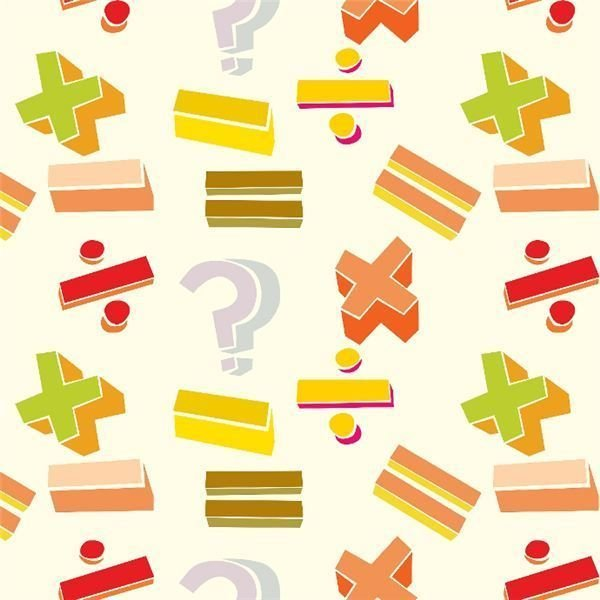# Multiplication and Division Lesson Plan: Solving for the Unknown Number in Equations

Lesson Objective: The lesson is aligned to the Common Core State Standards for Mathematics –

3.OA.4 Operations and Algebraic Thinking: Determine the unknown whole number in a multiplication or division equation relating three whole numbers.

Materials Required: arrays, blocks, books, triangles

## Lesson ProcedureBegin the lesson by working through some examples and walking students through the logical process they would use to solve the problems.

Example 1: Solve for the unknown in the equation 3 x ? = 12.

Have students think of the three numbers that relate. Encourage them to recognize that 3, 4, and 12 relate because 3 x 4 = 12. Discuss the 4 number sentences that relate to 3, 4, and 12:

• 3 x 4 = 12
• 4 x 3 = 12
• 12 / 3 = 4
• 12 / 4 = 3

Example 2: Solve for the unknown in the equation ? x 5 = 15.

Have students think of the three numbers that relate. Encourage them to recognize that 3, 5, and 15 relate because 3 x 5 = 15. Discuss the 4 number sentences that relate to 3, 5, and 15:

• 3 x 5 = 15
• 5 x 3 = 15
• 15 / 5 = 3
• 15 / 3 = 5

Example 3: Solve for the unknown in the equation 8 / ? = 4.

Have students think of the three numbers that relate. Encourage them to recognize that 2, 4, and 8 relate because 2 x 4 = 8. Discuss the 4 number sentences that relate to 2, 4, and 8:

• 2 x 4 = 8
• 4 x 2 = 8
• 8 / 2 = 4
• 8 / 4 = 2

Example 4: Solve for the unknown in the equation 35 / ? = 5.

Have students think of the three numbers that relate. Encourage them to recognize that 5, 7, and 35 relate because 5 x 7 = 35. Discuss the 4 number sentences that relate to 5, 7, and 35:

• 5 x 7 = 35
• 7 x 5 = 35
• 35 / 5 = 7
• 35 / 7 = 5

## Individual or Group Work

Find the unknown number that makes the equation true in each of the following equations.

1. 7 x 8 = ?

2. 4 x ? = 24

3. 32 / ? = 4

4. ? / 10 = 7

5. ? x 3 = 33

6. 2 x 5 = ?

7. 3 x ? = 18

8. ? x 4 = 28

9. ? / 3 = 3

10. 20 / 5 = ?

1. 56

2. 6

3. 8

4. 70

5. 11

6. 10

7. 6

8. 7

9. 9

10. 4

Goal: Students should be able to demonstrate the ability to solve for an unknown in multiplication and division equations by recognizing the three related numbers.

## This post is part of the series: Introducing Multiplication and Division in 3rd Grade Math

The activities in these lesson plans are designed to help your students understand the basic facts of multiplication and division, and relate them to problems that involve finding the number of objects in a group.